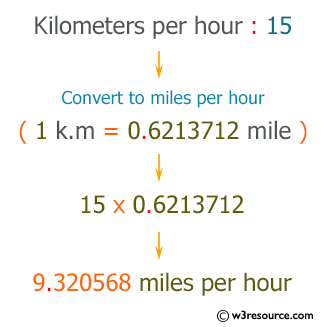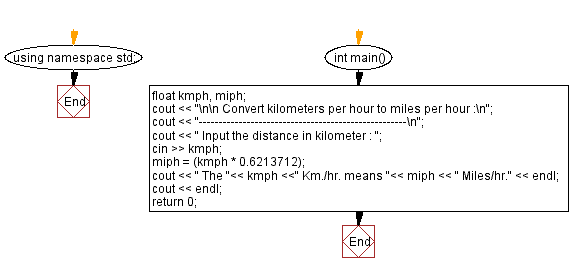﻿ C++ Exercises: Converts kilometers per hour to miles per hour - w3resource

# C++ Exercises: Converts kilometers per hour to miles per hour

## C++ Basic: Exercise-23 with Solution

Write a program in C++ that converts kilometers per hour to miles per hour.

Pictorial Presentation:Sample Solution:

C++ Code :

``````#include <iostream>
using namespace std;

int main()
{
float kmph, miph;
cout << "\n\n Convert kilometers per hour to miles per hour :\n";
cout << "----------------------------------------------------\n";
cout << " Input the distance in kilometer : ";
cin >> kmph;
miph = (kmph * 0.6213712);
cout << " The "<< kmph <<" Km./hr. means "<< miph << " Miles/hr." << endl;
cout << endl;
return 0;
}
``````

Sample Output:

``` Convert kilometers per hour to miles per hour :
----------------------------------------------------
Input the distance in kilometer : 25
The 25 Km./hr. means 15.5343 Miles/hr.
```

Flowchart:C++ Code Editor:

What is the difficulty level of this exercise?

﻿

## C++ Programming: Tips of the Day

Why is there no std::stou?

The most pat answer would be that the C library has no corresponding "strtou", and the C++11 string functions are all just thinly veiled wrappers around the C library functions: The std::sto* functions mirror strto*, and the std::to_string functions use sprintf.

Ref: https://bit.ly/3wtz2qA

We are closing our Disqus commenting system for some maintenanace issues. You may write to us at reach[at]yahoo[dot]com or visit us at Facebook